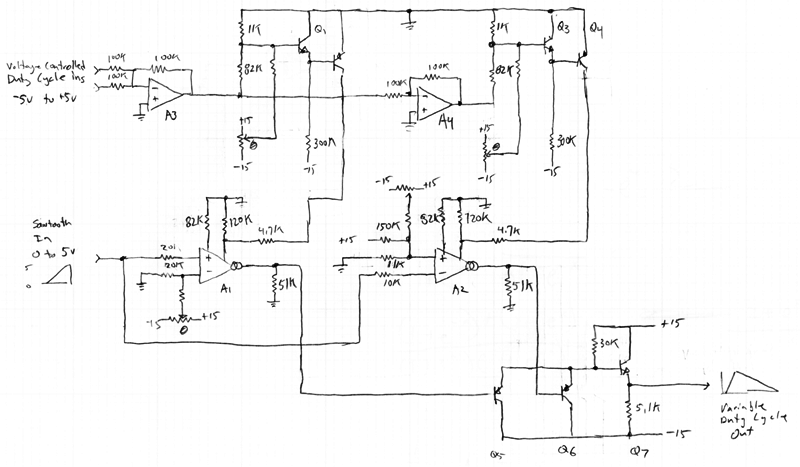# Voltage Controlled Duty Cycle Sawtooth Circuit

J. Donald Tillman
4 August 1999

There was a discussion on synth-diy a while ago about the problems of building a voltage controlled oscillator with a sawtooth wave output whose duty cycle (the ratio of ramp-up time to ramp-down time) is adjustable with a seperate control voltage.

This is a very hastily written description of my proposal for a circuit that will implement this by warping the waveform of a regular sawtooth wave VCO.

[Don: When you get around to it, talk about the musical advantages of such a thing, morphing, the harmonic spectra, the way to build a potentiometer controlled variable duty cycle oscillator, why you can't just integrate a PWM square wave, and so forth.]

In the following schematic, the input on the left is an audio frequency rising sawtooth wave going from 0.0V to +5.0V.  The input on the upper left is a duty cycle control voltage going from -5.0V (falling sawtooth) through 0.0V (triangle wave) to +5.0V (rising sawtooth).The power supply is +/- 15.0V. A1 and A2 are CA3280 OTAs. A3 and A4 are LF412 op amps.  The transistors are reasonable high beta NPNs and PNPs.  The values for the trimming circuitry is intentionally left off.

The basic idea is to take our rising sawtooth source and it's inverse, a falling sawtooth from +5.0V to 0.0V, adjust the relative gains of those two, and use the arithmetic minimum of those two signals as the output.  The gains would ideally go from 1.0 to infinity, but for practical reasons I've limited the range to 1.11 through 10.  If the two gains are equal to 2.0 the output will be a triangle wave.

To keep the output amplitude constant the two gains should be such that the sum of the recipricals of the gains is constant.  And if the output amplitude is going to be the same as the input amplitude, that constant value needs to be unity.

To do this I rely on the equation:

1/(1 + ex) + 1/(1 + e-x) = 1

For this application, x is proportional to the duty cycle control voltage, centered around zero for a 50% duty cycle.  1 + ex is proportional to one of the gains and 1 + e-x is proportional to the other.  The ex and e-x are implemented with simple ARP-style exponential circuits, and the 1s in the denominators are currents from resistors.

The signal path starts with the given sawtooth wave.  A1 and A2 are set up run at 0.24 mA for a 50% duty cycle; we need plenty of headroom to increase this gain, but the current for the lowest gain still needs to drive the output to full amplitude.

The 82k resistors set the OTAs' input diode current to 170uA, for a diode resistance of 416 ohms, for an input attenuation of 1/100th, easily accomodating the input amplitude.  From here, an OTA bias current of 0.24mA (triangle wave) and the 51k ohm output resistor will set the total gain to 2.0.

Transistors Q5 and Q6 are emitter followers with the lowest voltage taking over.  The output emitter follower compensates for the offset voltage of the previous transistor two.  Not exactly, but close enough for now.

Op amp A3 creates mixes the input control voltages, op amp A4 provides the negative. Both of these go to the ARP-style exponential circuits.  Adjust them to idle at 0.12mA each.

The 120kohm resistors are responsible for the 1s in the denominators of the equation and the 4.7kohm resistors limit the control current into the OTAs to a reasonble value.

No I haven't tested it yet.  This is only a sketch and I'm only publishing it here only to present it to the folks on synth-diy.

Correction: The resistor values on the inputs of A2 are wrong. They should be, top to bottom, 62k, 39k, and 20k.  They are supposed to bias A2's input so the sawtooth effectively ramps down from 5.0 volts.

-- Don